# Problem 56428. Easy Sequences 79: Trailing Zeros of Fibonorial Numbers at any Base

The fibonorial of an integer n is defined as follows:where: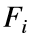is the i-th Fibonacci number (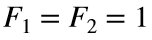and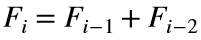for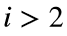).
In other words, the fibonorial of n,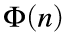, is the product of all Fibonacci numbers from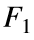to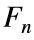.
Given an integer n and a number base b, write a function that calculates the number of trailing zeros of the fibonorial of n when written in base-b.
For example, for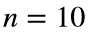and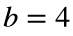, the function should return 2, because:
>> dec2base(prod([1 1 2 3 5 8 13 21 34 55]),4)
>> '13103120230200'
Forand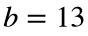, the function should return 1.
>> dec2base(prod([1 1 2 3 5 8 13 21 34 55]),13)
>> '1C4CB080'
--------------------
Hint: As a practice, you may want to solve first, the previous problem: Easy Sequences 78: Trailing Zeros of Factorial Numbers at any Base.

### Solution Stats

75.0% Correct | 25.0% Incorrect
Last Solution submitted on Feb 19, 2023

### Community Treasure Hunt

Find the treasures in MATLAB Central and discover how the community can help you!

Start Hunting!